MA17xexam01pdfLoading previews...
 First order linear differential equation Text Download (13kB)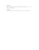First order linear differential equation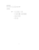multiplication of matrices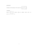determinant of matrices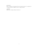conditions on a system of simultaneous equations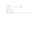partial differentiation
37 files in this resource

MA17xexam01pdf

Exam questions and solutions in PDFView Item